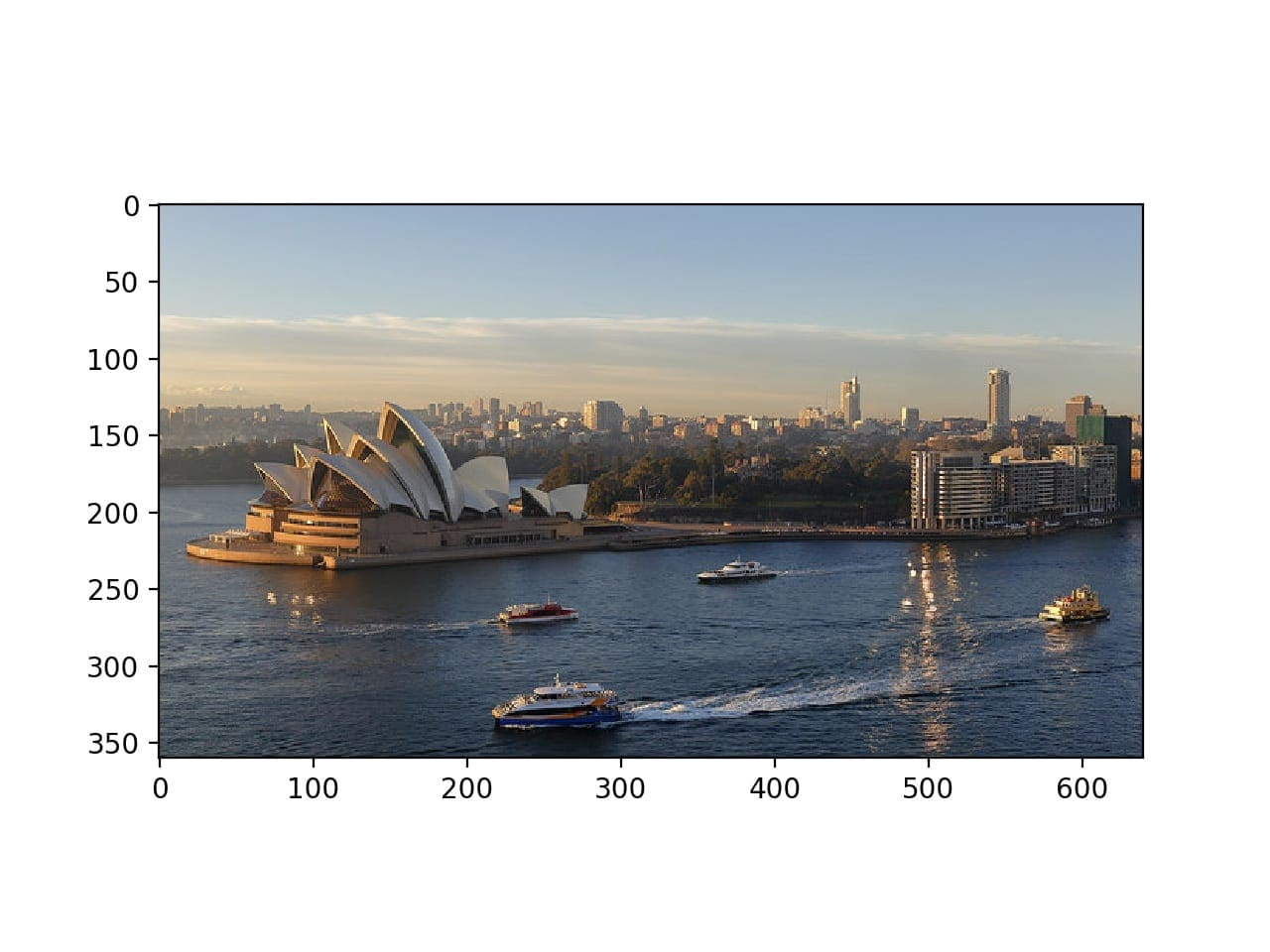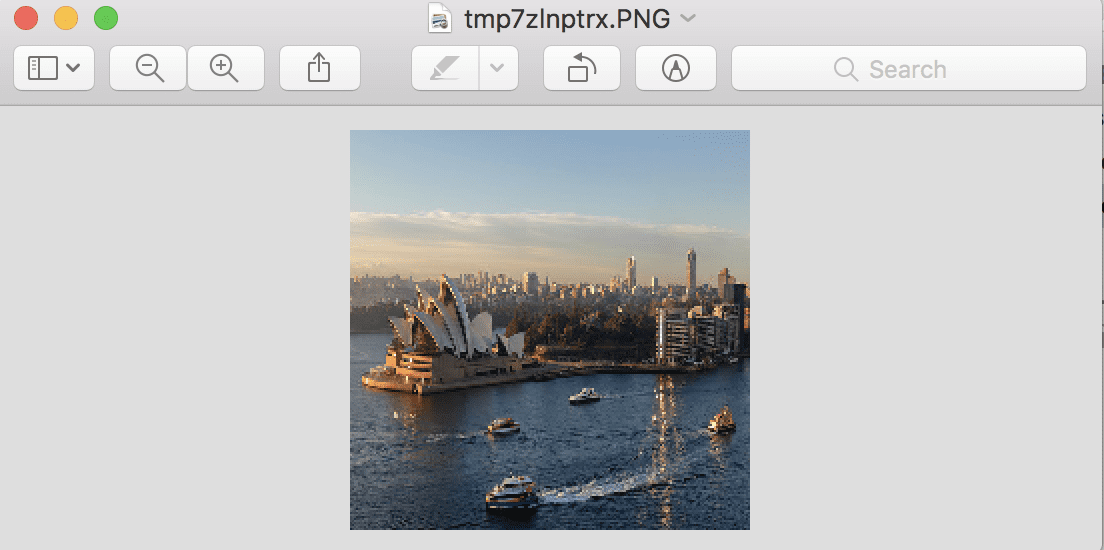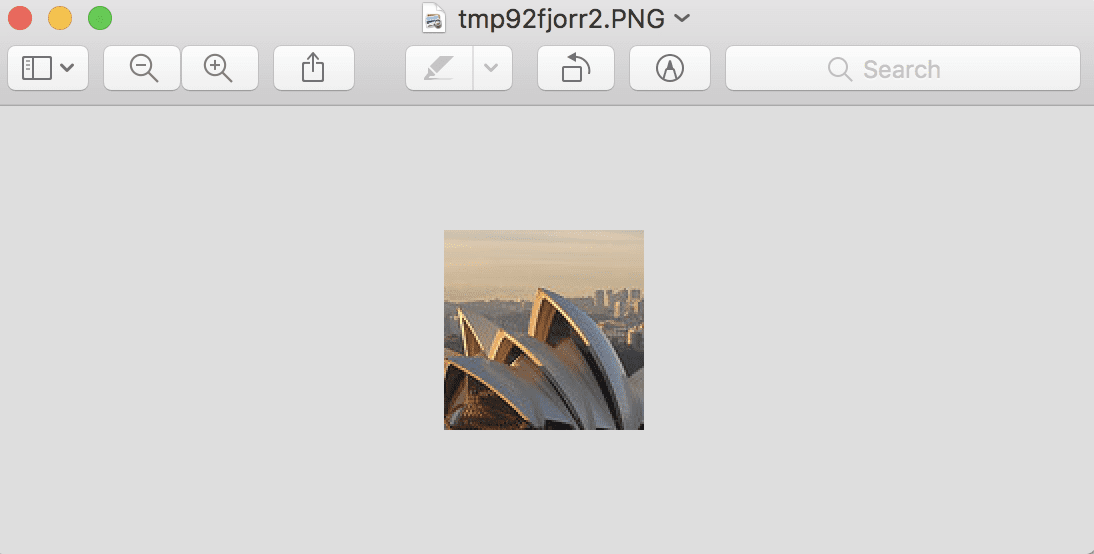# How to Load and Manipulate Images for Deep Learning in Python With PIL/Pillow

Source: Machine Learning Mastery

Before you can develop predictive models for image data, you must learn how to load and manipulate images and photographs.

The most popular and de facto standard library in Python for loading and working with image data is Pillow. Pillow is an updated version of the Python Image Library, or PIL, and supports a range of simple and sophisticated image manipulation functionality. It is also the basis for simple image support in other Python libraries such as SciPy and Matplotlib.

In this tutorial, you will discover how to load and manipulate image data using the Pillow Python library.

After completing this tutorial, you will know:

• How to install the Pillow library and confirm it is working correctly.
• How to load images from file, convert loaded images to NumPy arrays, and save images in new formats.
• How to perform basic transforms to image data such as resize, flips, rotations, and cropping.

Let’s get started.

## Tutorial Overview

This tutorial is divided into six parts; they are:

1. How to Install Pillow
2. How to Load and Display Images
3. How to Convert Images to NumPy Arrays and Back
4. How to Save Images to File
5. How to Resize Images
6. How to Flip, Rotate, and Crop Images

## How to Install Pillow

The Python Imaging Library, or PIL for short, is an open source library for loading and manipulating images.

It was developed and made available more than 25 years ago and has become a de facto standard API for working with images in Python. The library is now defunct and no longer updated and does not support Python 3.

Pillow is a PIL library that supports Python 3 and is the preferred modern library for image manipulation in Python. It is even required for simple image loading and saving in other Python scientific libraries such as SciPy and Matplotlib.

The Pillow library is installed as a part of most SciPy installations; for example, if you are using Anaconda.

For help setting up your SciPy environment, see the step-by-step tutorial:

If you manage the installation of Python software packages yourself for your workstation, you can easily install Pillow using pip; for example:

`sudo pip install Pillow`

For more help installing Pillow manually, see:

Pillow is built on top of the older PIL and you can confirm that the library was installed correctly by printing the version number; for example:

```# check PIL and Pillow version numbers
import PIL
print('Pillow Version:', PIL.__version__)
print('PIL Version:', PIL.VERSION)```

Running the example will print the version numbers for PIL and Pillow; your version numbers should be the same or higher.

```Pillow Version: 5.3.0
PIL Version: 1.1.7```

Now that your environment is set up, let’s look at how to load an image.

## How to Load and Display Images

We need a test image to demonstrate some important features of using the Pillow library.

In this tutorial, we will use a photograph of the Sydney Opera House, taken by Ed Dunens and made available on Flickr under a creative commons license, some rights reserved.Sydney Opera House

Images are typically in PNG or JPEG format and can be loaded directly using the open() function on Image class. This returns an Image object that contains the pixel data for the image as well as details about the image. The Image class is the main workhorse for the Pillow library and provides a ton of properties about the image as well as functions that allow you to manipulate the pixels and format of the image.

The ‘format‘ property on the image will report the image format (e.g. JPEG), the ‘mode‘ will report the pixel channel format (e.g. RGB or CMYK), and the ‘size‘ will report the dimensions of the image in pixels (e.g. 640×480).

The show() function will display the image using your operating systems default application.

The example below demonstrates how to load and show an image using the Image class in the Pillow library.

```# load and show an image with Pillow
from PIL import Image
image = Image.open('opera_house.jpg')
# summarize some details about the image
print(image.format)
print(image.mode)
print(image.size)
# show the image
image.show()```

Running the example will first load the image, report the format, mode, and size, then show the image on your desktop.

```JPEG
RGB
(640, 360)```

The image is shown using the default image preview application for your operating system, such as Preview on MacOS.Sydney Opera House Displayed Using the Default Image Preview Application

Now that you know how to load an image, let’s look at how you can access the pixel data of images.

## How to Convert Images to NumPy Arrays and Back

Often in machine learning, we want to work with images as NumPy arrays of pixel data.

With Pillow installed, you can also use the Matplotlib library to load the image and display it within a Matplotlib frame.

This can be achieved using the imread() function that loads the image an array of pixels directly and the imshow() function that will display an array of pixels as an image.

The example below loads and displays the same image using Matplotlib that, in turn, will use Pillow under the covers.

```# load and display an image with Matplotlib
from matplotlib import image
from matplotlib import pyplot
# load image as pixel array
# summarize shape of the pixel array
print(data.dtype)
print(data.shape)
# display the array of pixels as an image
pyplot.imshow(data)
pyplot.show()```

Running the example first loads the image and then reports the data type of the array, in this case, 8-bit unsigned integers, then reports the shape of the array, in this case, 360 pixels wide by 640 pixels high and three channels for red, green, and blue.

```uint8
(360, 640, 3)```

Finally, the image is displayed using Matplotlib.Sydney Opera House Displayed Using Matplotlib

The Matplotlib wrapper functions can be more effective than using Pillow directly.

Nevertheless, you can access the pixel data from a Pillow Image. Perhaps the simplest way is to construct a NumPy array and pass in the Image object. The process can be reversed converting a given array of pixel data into a Pillow Image object using the Image.fromarray() function. This can be useful if image data is manipulated as a NumPy array and you then want to save it later as a PNG or JPEG file.

The example below loads the photo as a Pillow Image object and converts it to a NumPy array, then converts it back to an Image object again.

```# load image and convert to and from NumPy array
from PIL import Image
from numpy import asarray
image = Image.open('opera_house.jpg')
# convert image to numpy array
data = asarray(image)
# summarize shape
print(data.shape)
# create Pillow image
image2 = Image.fromarray(data)
# summarize image details
print(image2.format)
print(image2.mode)
print(image2.size)```

Running the example first loads the photo as a Pillow image then converts it to a NumPy array and reports the shape of the array. Finally, the array is converted back into a Pillow image and the details are reported.

```(360, 640, 3)
JPEG
RGB
(640, 360)```

Both approaches are effective for loading image data into NumPy arrays, although the Matplotlib imread() function uses fewer lines of code than loading and converting a Pillow Image object and may be preferred.

For example, you could easily load all images in a directory as a list as follows:

```# load all images in a directory
from os import listdir
from matplotlib import image
# load all images in a directory
for filename in listdir('images'):
print('> loaded %s %s' % (filename, img_data.shape))```

Now that we know how to load images as NumPy arrays, let’s look at how to save images to file.

## How to Save Images to File

An image object can be saved by calling the save() function.

This can be useful if you want to save an image in a different format, in which case the ‘format‘ argument can be specified, such as PNG, GIF, or PEG.

For example, the code listing below loads the photograph in JPEG format and saves it in PNG format.

```# example of saving an image in another format
from PIL import Image
image = Image.open('opera_house.jpg')
# save as PNG format
image.save('opera_house.png', format='PNG')
# load the image again and inspect the format
image2 = Image.open('opera_house.png')
print(image2.format)```

Running the example loads the JPEG image, saves it in PNG format, then loads the newly saved image again, and confirms that the format is indeed PNG.

`PNG`

Saving images is useful if you perform some data preparation on the image before modeling. One example is converting color images (RGB channels) to grayscale (1 channel).

There are a number of ways to convert an image to grayscale, but Pillow provides the convert() function and the mode ‘L‘ will convert an image to grayscale.

```# example of saving a grayscale version of a loaded image
from PIL import Image
image = Image.open('opera_house.jpg')
# convert the image to grayscale
gs_image = image.convert(mode='L')
# save in jpeg format
gs_image.save('opera_house_grayscale.jpg')
# load the image again and show it
image2 = Image.open('opera_house_grayscale.jpg')
# show the image
image2.show()```

Running the example loads the photograph, converts it to grayscale, saves the image in a new file, then loads it again and shows it to confirm that the photo is now grayscale instead of color.Example of Grayscale Version of Photograph

## How to Resize Images

It is important to be able to resize images before modeling.

Sometimes it is desirable to thumbnail all images to have the same width or height. This can be achieved with Pillow using the thumbnail() function. The function takes a tuple with the width and height and the image will be resized so that the width and height of the image are equal or smaller than the specified shape.

For example, the test photograph we have been working with has the width and height of (640, 360). We can resize it to (100, 100), in which case the largest dimension, in this case, the width, will be reduced to 100, and the height will be scaled in order to retain the aspect ratio of the image.

The example below will load the photograph and create a smaller thumbnail with a width and height of 100 pixels.

```# create a thumbnail of an image
from PIL import Image
image = Image.open('opera_house.jpg')
# report the size of the image
print(image.size)
# create a thumbnail and preserve aspect ratio
image.thumbnail((100,100))
# report the size of the thumbnail
print(image.size)```

Running the example first loads the photograph and reports the width and height. The image is then resized, in this case, the width is reduced to 100 pixels and the height is reduced to 56 pixels, maintaining the aspect ratio of the original image.

```(640, 360)
(100, 56)```

We may not want to preserve the aspect ratio, and instead, we may want to force the pixels into a new shape.

This can be achieved using the resize() function that allows you to specify the width and height in pixels and the image will be reduced or stretched to fit the new shape.

The example below demonstrates how to resize a new image and ignore the original aspect ratio.

```# resize image and force a new shape
from PIL import Image
image = Image.open('opera_house.jpg')
# report the size of the image
print(image.size)
# resize image and ignore original aspect ratio
img_resized = image.resize((200,200))
# report the size of the thumbnail
print(img_resized.size)```

Running the example loads the image, reports the shape of the image, then resizes it to have a width and height of 200 pixels.

```(640, 360)
(200, 200)```

The sized of the image is shown and we can see that the wide photograph has been compressed into a square, although all of the features are still quite visible and obvious.

Standard resampling algorithms are used to invent or remove pixels when resizing, and you can specify a technique, although default is a bicubic resampling algorithm that suits most general applications.Resized Photograph That Does Not Preserve the Original Aspect Ratio

## How to Flip, Rotate, and Crop Images

Simple image manipulation can be used to create new versions of images that, in turn, can provide a richer training dataset when modeling.

Generally, this is referred to as data augmentation and may involve creating flipped, rotated, cropped, or other modified versions of the original images with the hope that the algorithm will learn to extract the same features from the image data regardless of where they might appear.

You may want to implement your own data augmentation schemes, in which case you need to know how to perform basic manipulations of your image data.

### Flip Image

An image can be flipped by calling the flip() function and passing in a method such as FLIP_LEFT_RIGHT for a horizontal flip or FLIP_TOP_BOTTOM for a vertical flip. Other flips are also available

The example below creates both horizontal and vertical flipped versions of the image.

```# create flipped versions of an image
from PIL import Image
from matplotlib import pyplot
image = Image.open('opera_house.jpg')
# horizontal flip
hoz_flip = image.transpose(Image.FLIP_LEFT_RIGHT)
# vertical flip
ver_flip = image.transpose(Image.FLIP_TOP_BOTTOM)
# plot all three images using matplotlib
pyplot.subplot(311)
pyplot.imshow(image)
pyplot.subplot(312)
pyplot.imshow(hoz_flip)
pyplot.subplot(313)
pyplot.imshow(ver_flip)
pyplot.show()```

Running the example loads the photograph and creates horizontal and vertical flipped versions of the photograph, then plots all three versions as subplots using Matplotlib.

You will note that the imshow() function can plot the Image object directly without having to convert it to a NumPy array.Plot of Original, Horizontal, and Vertical Flipped Versions of a Photograph

### Rotate Image

An image can be rotated using the rotate() function and passing in the angle for the rotation.

The function offers additional control such as whether or not to expand the dimensions of the image to fit the rotated pixel values (default is to clip to the same size), where to center the rotation the image (default is the center), and the fill color for pixels outside of the image (default is black).

The example below creates a few rotated versions of the image.

```# create rotated versions of an image
from PIL import Image
from matplotlib import pyplot
image = Image.open('opera_house.jpg')
# plot original image
pyplot.subplot(311)
pyplot.imshow(image)
# rotate 45 degrees
pyplot.subplot(312)
pyplot.imshow(image.rotate(45))
# rotate 90 degrees
pyplot.subplot(313)
pyplot.imshow(image.rotate(90))
pyplot.show()```

Running the example plots the original photograph, then a version of the photograph rotated 45 degrees, and another rotated 90 degrees.

You can see that in both rotations, the pixels are clipped to the original dimensions of the image and that the empty pixels are filled with black color.Plot of Original and Rotated Version of a Photograph

### Cropped Image

An image can be cropped: that is, a piece can be cut out to create a new image, using the crop() function.

The crop function takes a tuple argument that defines the two x/y coordinates of the box to crop out of the image. For example, if the image is 2,000 by 2,000 pixels, we can clip out a 100 by 100 box in the middle of the image by defining a tuple with the top-left and bottom-right points of (950, 950, 1050, 1050).

The example below demonstrates how to create a new image as a crop from a loaded image.

```# example of cropping an image
from PIL import Image
image = Image.open('opera_house.jpg')
# create a cropped image
cropped = image.crop((100, 100, 200, 200))
# show cropped image
cropped.show()```

Running the example creates a cropped square image of 100 pixels starting at 100,100 and extending down and left to 200,200. The cropped square is then displayed.Example of a Cropped Version of a Photograph

## Extensions

This section lists some ideas for extending the tutorial that you may wish to explore.

• Your Own Images. Experiment with Pillow functions for reading and manipulating images with your own image data.
• More Transforms. Review the Pillow API documentation and experiment with additional image manipulation functions.
• Image Pre-processing. Write a function to create augmented versions of an image ready for use with a deep learning neural network.

If you explore any of these extensions, I’d love to know.

This section provides more resources on the topic if you are looking to go deeper.

## Summary

In this tutorial, you discovered how to load and manipulate image data using the Pillow Python library.

Specifically, you learned:

• How to install the Pillow library and confirm it is working correctly.
• How to load images from file, convert loaded images to NumPy arrays, and save images in new formats.
• How to perform basic transforms to image data such as resize, flips, rotations, and cropping.

Do you have any questions?